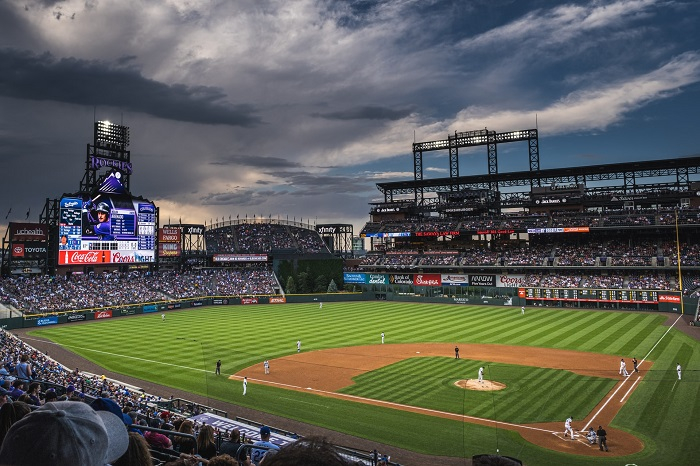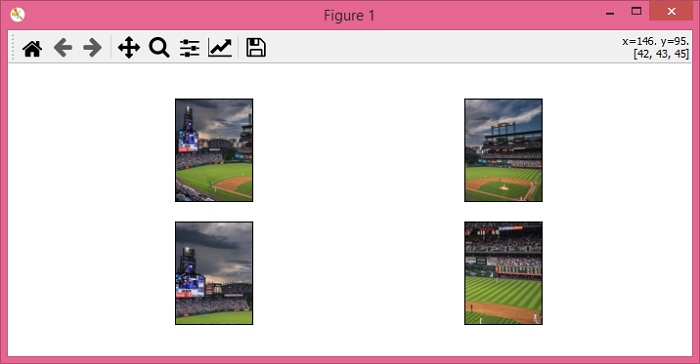# PyTorch – torchvision.transforms – RandomResizedCrop()

PyTorchServer Side ProgrammingProgramming

RandomResizedCrop() transform crops a random area of the original input image. This crop size is randomly selected and finally the cropped image is resized to the given size. RandomResizedCrop() transform is one of the transforms provided by the torchvision.transforms module. This module contains many important transforms that can be used to perform different types of manipulations on the image data.

RandomResizedCrop() accepts both PIL and tensor images. A tensor image is a PyTorch tensor with shape [..., H, W], where ... means a number of dimensions, H is the image height, and W is the image width. If the image is neither a PIL image nor a tensor image, then we first convert it to a tensor image and then apply the transform.

## Syntax

torchvision.transforms.RandomResizedCrop(size)(img)

where size is the desired crop size. size is a sequence like (h, w), where h and w are the height and width of the cropped image. If size is an int, the cropped image is a square image.

It returns the cropped image resized with a given size.

## Steps

We could use the following steps to crop a random portion of an input image and resize it to given size −

• Import the required libraries. In all the following examples, the required Python libraries are torch, Pillow, and torchvision. Make sure you have already installed them.

import torch
import torchvision
import torchvision.transforms as T
from PIL import Image
import matplotlib.pyplot as plt
• Read the input image. The input image is a PIL image or a torch tensor of shape [..., H, W].

img = Image.open('baseball.png')
• Define a transform to crop a random portion on the input image and then resize to given size. Here given size is (150,250) for rectangular crop and 250 for square crop. Change the crop size according your need.

# transform for rectangular crop
transform = T.RandomResizedCrop((150,250))
# transform for square crop
transform = T.RandomResizedCrop(250)
• Apply the above-defined transform on the input image to crop a random portion on the input image and then resize it to given size.

cropped_img = transform(img)
• Show the cropped image and then the resized image

cropped_img.show()

## Input Image

This image is used as the input in all the following examples.## Example 1

In this program, the random portion of the input image is cropped and then resized with the size (150, 250).

# import required libraries
import torch
import torchvision.transforms as T
from PIL import Image
import matplotlib.pyplot as plt

# read the input image
img = Image.open('baseball.png')

# define a transform to crop a random portion of an image
# and resize it to given size
transform = T.RandomResizedCrop(size=(350,600))

# apply above defined transform to the input image
img = transform(img)

# display the cropped image
img.show()

## Output

It will produce the following output −## Example 2

import torch
import torchvision.transforms as T
from PIL import Image
import matplotlib.pyplot as plt

img = Image.open('baseball.png')
transform = T.RandomResizedCrop(size = (200,150), scale=(0.08,
1.0), ratio=(0.75, 1.3333333333333333))
imgs = [transform(img) for _ in range(4)]
fig = plt.figure(figsize=(7,3))
rows, cols = 2,2
for j in range(0, len(imgs)):
plt.show()